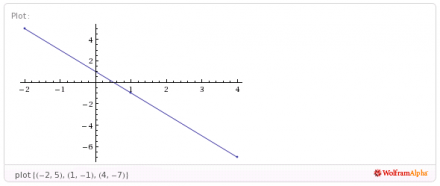If the function 2x + y = 1 has the domain {-2, 1, 4}, then what is the corresponding range? A. {5, -3, -7} B. {5, 1, 7} C. {5, -1, -7} D. {-3, -1, 9}

Solve for y.
2x + y = 1
y = 1 - 2x    (subtract 2x)

Substitute for x
y = 1 - 2{-2, 1, 4) = 1 + {4, -2, -8} = {5, -1, -7}
Answer (C) seems to match.
_____
You can do the substitution one number at a time, but sometimes it is convenient just to do them all at once.thanked the writer.